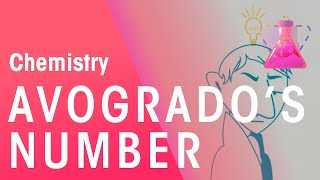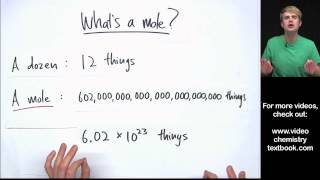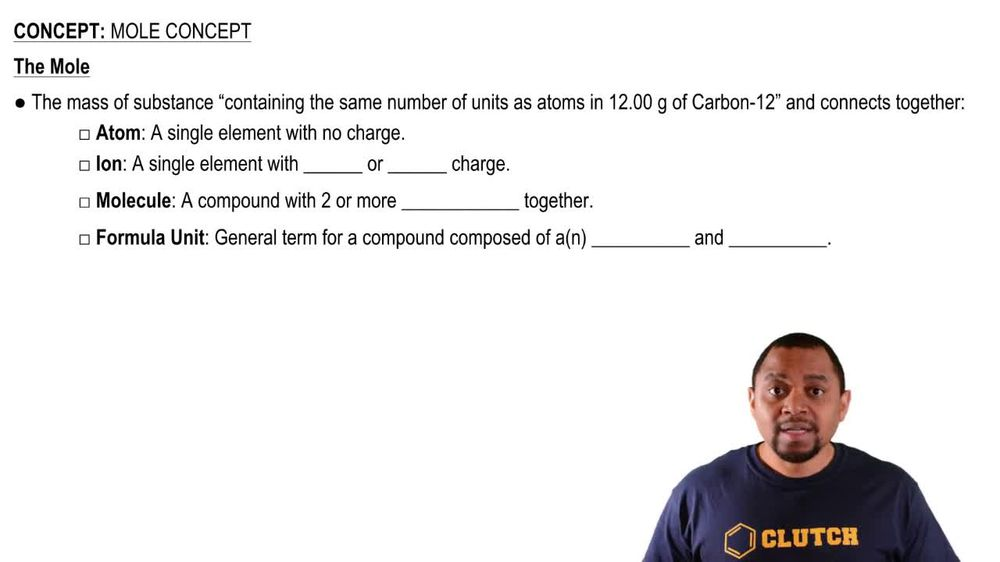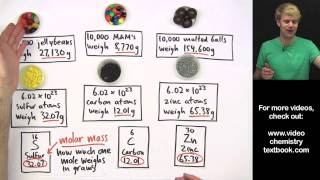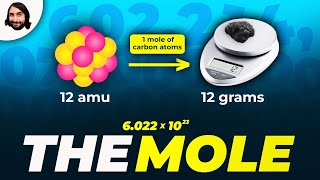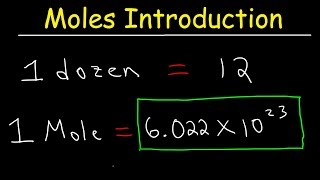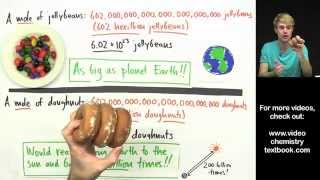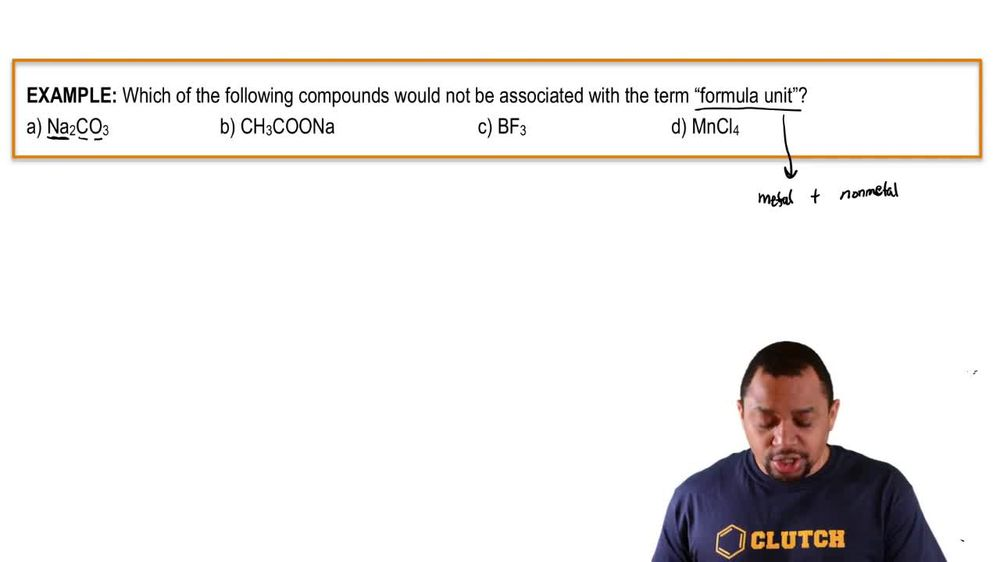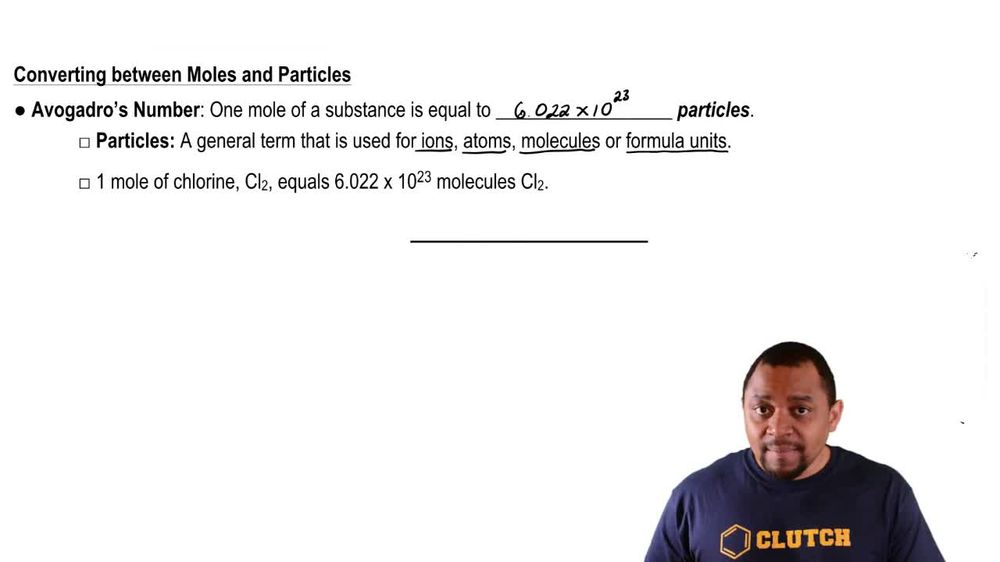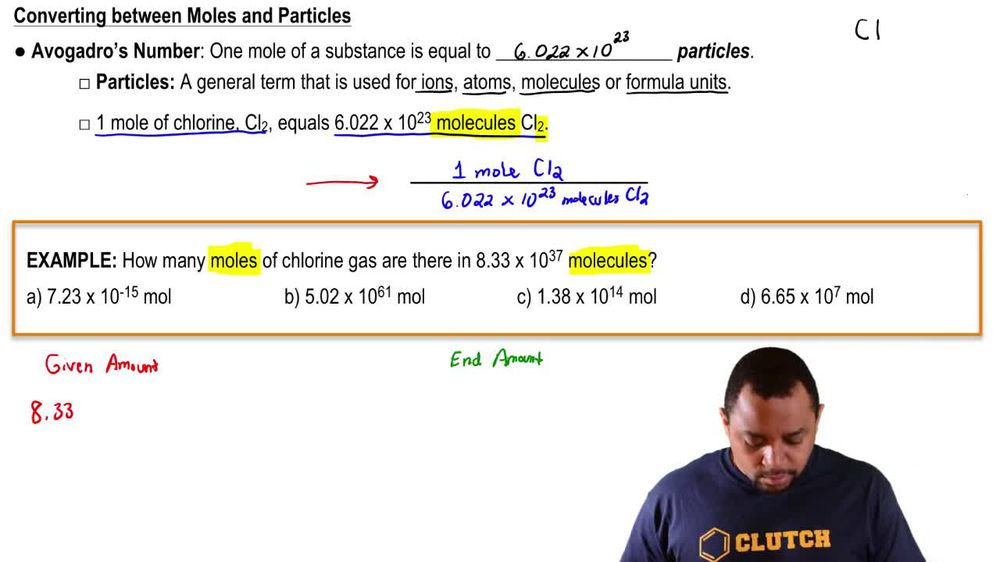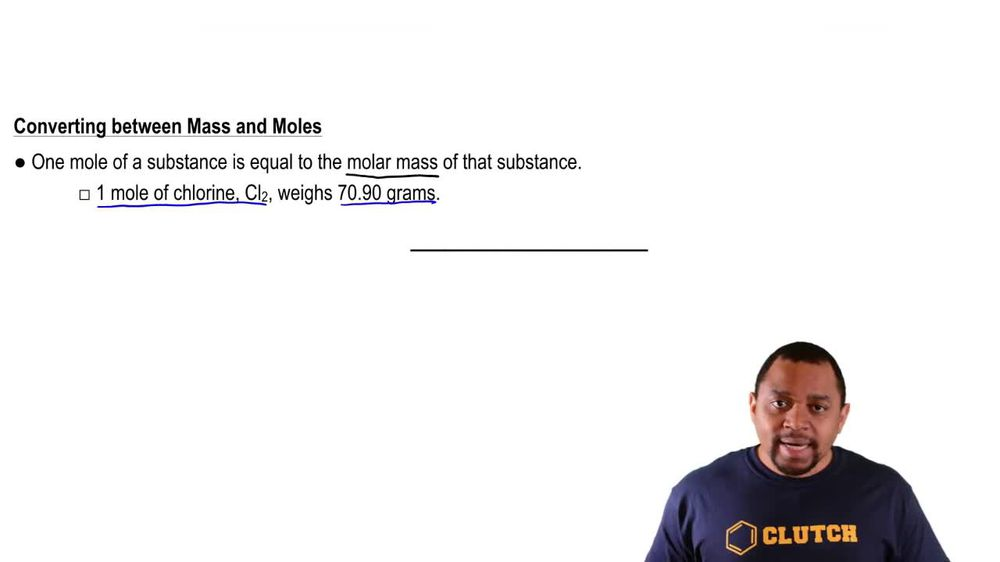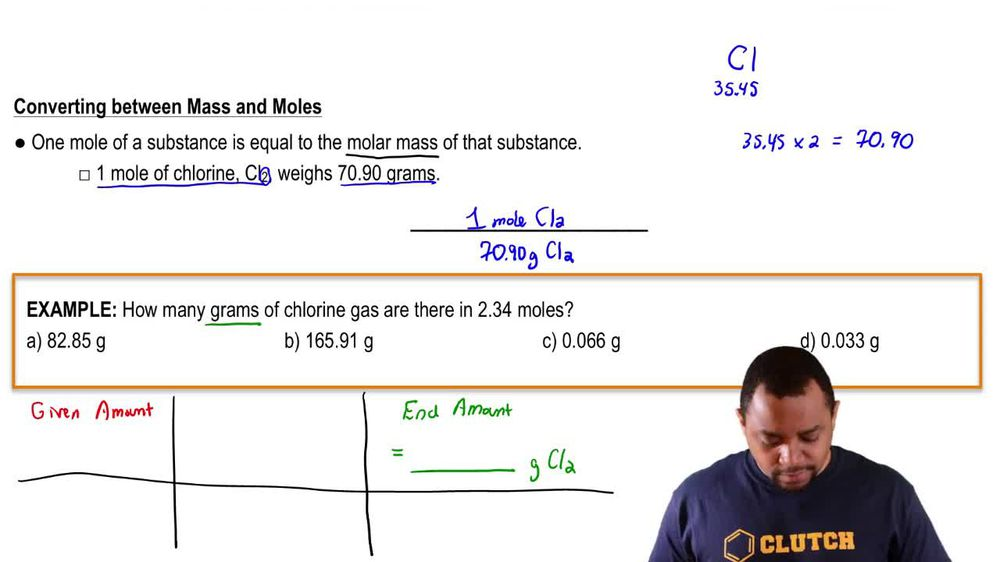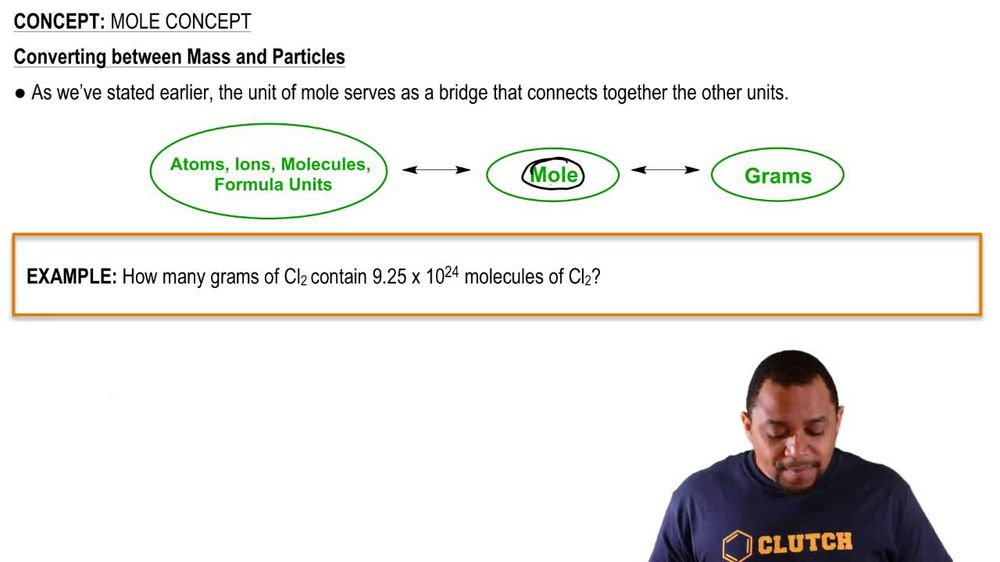Start typing, then use the up and down arrows to select an option from the list.
1. 2. Atoms & Elements2. Mole Concept
Problem

# What is the mass of 4.91 * 1021 platinum atoms?

Relevant Solution1m
Play a video:
Hey everyone. Our question here is asking us, how much do 6.2 times 10 to the 21st atoms of tungsten weight in g. To answer this question we're first going to meet to look at our periodic table and get the molar mass of tungsten. Now looking at our periodic table, we see that we have 183 0.84 g of tungsten per one mole of tungsten. So using that into our question, We're first going to take our 6.2 times 10 to the 21st atoms of tungsten and we're going to use avocados number to convert from Adams into moles. So we know that we have 6.02, 2 times 10 to the 23rd Adams of Tungsten per one mole of Tungsten plugging in our molar mass, We found that we have 183.84 g of tungsten per one mole of Tungsten. Now, when we calculate this out and cancel out our units, we end up with a value of 1. grams of truxton. And this is going to be our final answer. So I hope this made sense. And let us know if you have any questions